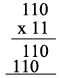SEARCH HOMEMath Central Quandaries & QueriesQuestion from Michael, a student: multiply in the indicated base 110two*11twoHi Michael,

$1 \times a = a$ for any number $a$ in any base and hence working in base twoAll that remains is to add the resulting two rows. Try it and if you have difficulty write back and tell us what you did.

PennyMath Central is supported by the University of Regina and The Pacific Institute for the Mathematical Sciences.